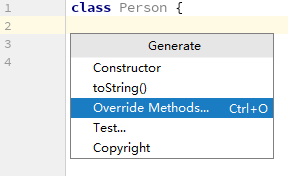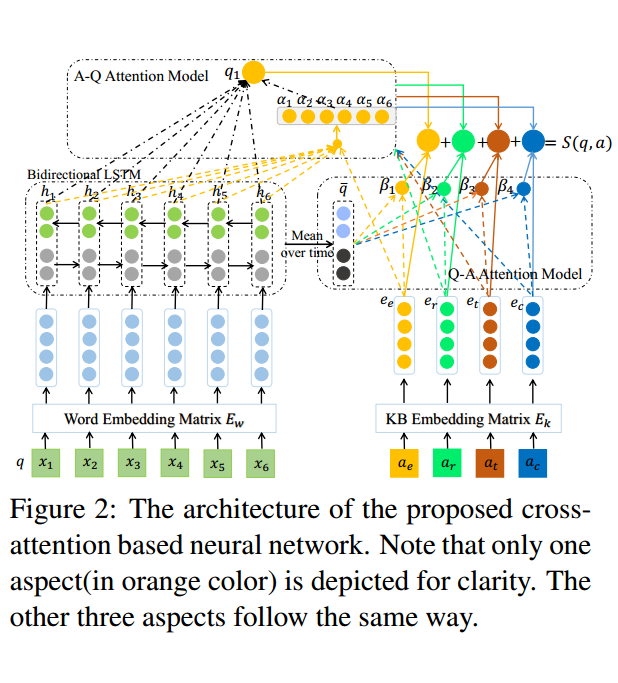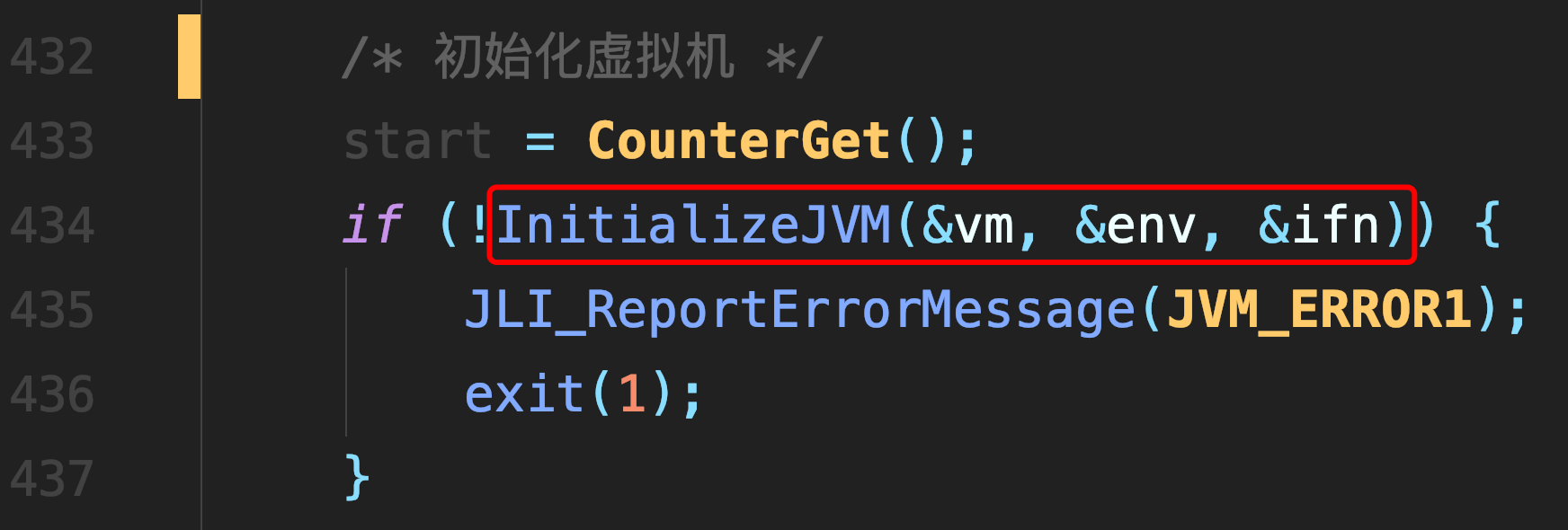# [转载] $CF171H$ 题解

$$m / 2^{2n-2}=0$$ 时，表示 $$m$$ 点在左下 $$n-1$$ 级城市中，根据 $$m$$ 点在 $$n-1$$ 级城市中的坐标 $$(x,y)$$ 顺时针旋转 $$90^\circ$$ 再垂直翻转可得 $$m$$ 点在 $$n$$ 级城市中的坐标 $$(y,x)$$

$$m / 2^{2n-2}=1$$ 时，表示 $$m$$ 点在左上 $$n-1$$ 级城市中，根据 $$m$$ 点在 $$n-1$$ 级城市中的坐标 $$(x,y)$$ 向上平移可得 $$m$$ 点在 $$n$$ 级城市中的坐标 $$(x,y+2^{n-1})$$

$$m / 2^{2n-2}=2$$ 时，表示 $$m$$ 点在右上 $$n-1$$ 级城市中，根据 $$m$$ 点在 $$n-1$$ 级城市中的坐标 $$(x,y)$$ 向右向上平移可得 $$m$$ 点在 $$n$$ 级城市中的坐标 $$(x+2^{n-1},y+2^{n-1})$$

$$m / 2^{2n-2}=3$$ 时，表示 $$m$$ 点在右下 $$n-1$$ 级城市中，根据 $$m$$ 点在 $$n-1$$ 级城市中的坐标 $$(x,y)$$ 顺时针旋转 $$90^\circ$$ 再垂直翻转然后水平翻转最后垂直翻转可得 $$m$$ 点在 $$n$$ 级城市中的坐标 $$(2^n-y-1,2^{n-1}-x-1)$$

$$trick$$ ：对于坐标的计算和转移可以使用如 $$pair<int,int>$$ 类型的函数，返回值需要 $$make \_ pair(x,y)$$

#include<bits/stdc++.h> using namespace std; inline int read() { int ret=0,f=1; char ch=getchar(); while(ch>'9'||ch<'0') { if(ch=='-') f=-1; ch=getchar(); } while(ch>='0'&&ch<='9') { ret=(ret<<1)+(ret<<3)+ch-'0'; ch=getchar(); } return ret*f; } int a,b,ansx,ansy; pair <int,int> calc(int n,int m) { if(n==0) return make_pair(0,0); int len=1<<(n-1),mod=1<<(2*n-2),cas,x,y; pair<int,int> tmp=calc(n-1,m%mod); x=tmp.first; y=tmp.second; cas=m/mod; if(cas==1) return make_pair(x,y+len); if(cas==2) return make_pair(x+len,y+len); if(cas==0) return make_pair(y,x); if(cas==3) return make_pair(len*2-y-1,len-x-1); } int main() { a=read(); b=read(); pair<int,int> ans; ans=calc(a,b); ansx=ans.first; ansy=ans.second; printf("%d %d\n",ansx,ansy); return 0; }

##### 热门推荐
•浏览(655)
•浏览(565)
•浏览(548)
•浏览(488)
•浏览(434)
•浏览(424)
•浏览(381)
•浏览(367)
•浏览(362)
•浏览(357)